## Logical Venn Diagram

#### Logical Venn Diagram

1. Which of the following diagrams represents Colour , Cloth and Merchant?

1. From above the given question , it is clear that
All the three are different from one another.

##### Correct Option: A

From above the given question , it is clear that
All the three are different from one another.
It is clear that option ( A ) represents the best relationship between Colour , Cloth and Merchant .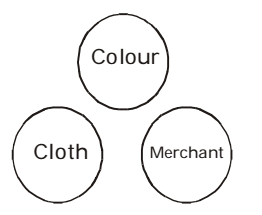1. Select from the given diagram the one that illustrates the relationship among the given 3 classes: children below 18, persons in the electoral list, those who have voted.

1. According to above given question , we can say that
children below 18 are different from persons in the electoral list and those who have voted.

##### Correct Option: A

According to above given question , we can say that
children below 18 are different from persons in the electoral list and those who have voted.
From above it is clear that option ( A ) represents the best relationship between children below 18, persons in the electoral list and those who have voted . As shown in given venn - diagram .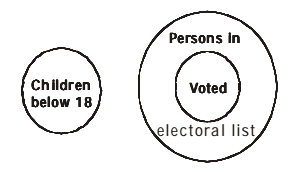1. Which one of the following represents Lime, Cement and Brick?

1. As per the above given question , we can say that
Lime, cement and Brick are three distinct items. Therefore, these can be shown by three disjoint circles.

##### Correct Option: C

As per the above given question , we can say that
Lime, cement and Brick are three distinct items. Therefore, these can be shown by three disjoint circles.
From above it is clear that option ( C ) represents the best relationship between Lime, Cement and Brick . As shown in given venn - diagram .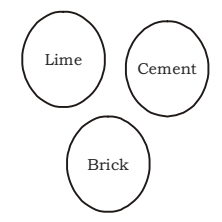1. Which of the following diagrams represents the relationship among Sun, Moon and Star?

1. As we know that
Sun is a star.
Moon is a satellite.

##### Correct Option: D

As we know that
Sun is a star.
Moon is a satellite.
Star will be a big circle and Sun will be a small circle under Star.
Moon is a satellite. So it will be a separate circle.
It shows that Sun, Moon and Star represents the relationship in given figure.
Therefore , required answer will be option ( D ) .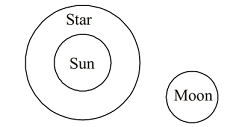1. In the following figure, how many educated people are employed?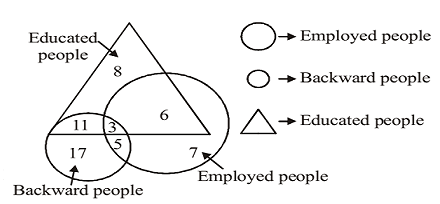1. As per the given above venn-diagram , we can say that
Total number of educated people = 3 + 6 = 9

##### Correct Option: D

As per the given above venn-diagram , we can say that
Total number of educated people = 3 + 6 = 9
Hence , 9 educated people are employed .